The and#8747;and#915;<sup>3<em>and#955;I</em></sup> Statistical Convergence of pre-Cauchy over the p- Metric Space Defined by Musielak Orlicz Function | OMICS International

# The ∫Γ3λI Statistical Convergence of pre-Cauchy over the p- Metric Space Defined by Musielak Orlicz Function

Deepmala1, Subramanian N2 and Lakshmi Narayan Mishra3*

1SQC and OR Unit, Indian Statistical Institute, Kolkata, West Bengal, India

2Department of Mathematics, SASTRA University, Thanjavur, India

3Department of Mathematics, National Institute of Technology, Silchar, Cachar, Assam, India

*Corresponding Author:
Lakshmi Narayan M
Department of Mathematics
National Institute of Technology
Silchar, District - Cachar, Assam, India
Tel: 03842 242 273
E-mail: [email protected]

Received Date: May 23, 2016; Accepted Date: September 01, 2016; Published Date: September 05, 2016

Citation: Deepmala, Subramanian N, Mishra LN (2016) The ∫Γ3λI Statistical Convergence of pre-Cauchy over the p- Metric Space Defined by Musielak Orlicz Function. Adv Robot Autom 5:155. doi: 10.4172/2168-9695.1000155

Copyright: © 2016 Deepmala, et al. This is an open-access article distributed under the terms of the Creative Commons Attribution License, which permits unrestricted use, distribution, and reproduction in any medium, provided the original author and source are credited.

Visit for more related articles at Advances in Robotics & Automation

#### Abstract

In this paper we are concerned with ∫Γ3λ I statistical convergence of pre-cauchy triple sequences. ∫Γ3λ I statistical convergence implies ∫Γ3λ I statistical pre-Cauchy condition and examine some properties of these concepts. We examine some properties of these concepts, if the triple entire sequence spaces is statistically convergent then statistically pre-Cauchy and also triple sequence of ideal (I3)- is statistically pre-Cauchy

#### Keywords

Analytic sequence; Double sequences; Γ3 space; Musielak - Orlicz function; p- metric space; Ideal; Filter; ∫Γ3λ I statistical convergence; ∫Γ3λ I statistical pre-Cauchy

#### Introduction

We introduce ∫Γ3λ I sequence space and also discuss ∫Γ3λ I is statistically convergent is pre-Cauchy and the ideal space is pre- Cauchy. Throughout w, x and Λ denote the classes of all, gai and analytic scalar valued single sequences, respectively. We write w3 for the set of all complex triple sequences (xmnk), where m, n, k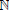the set of positive integers. Then, w3 is a linear space under the coordinate wise addition and scalar multiplication. We can represent triple sequences by matrix. In case of double sequences we write in the form of a square. In the case of a triple sequence it will be in the form of a box in three dimensional case.

Some initial work on double series and interesting results are found in Apostol  and double sequence spaces is found in Hardy , Subramanian et al. , Deepmala et al. [4-7], Mishra et al. [8,9], Mishra and Mishra , Mishra  and many others. Later on investigated by some initial work on triple sequence spaces is found in sahiner et al. , Esi et al. [12-15], Savas et al.  , Subramanian et al. , Prakash et al. [18,19] and many others.

Let (xmnk) be a triple sequence of real or complex numbers. Then the series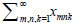is called a triple series. The triple seriesgive one space is said to be convergent if and only if the triple sequence (Smnk) is convergent, where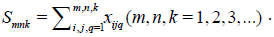A sequence x = (xmnk) is said to be triple analytic if;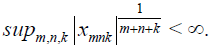The vector space of all triple analytic sequences are usually denoted by Λ3. A sequence x = (xmnk) is called triple entire sequence if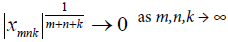The vector space of all triple entire sequences are usually denoted by Γ3. The space Λ3 and Γ3 is a metric space with the metric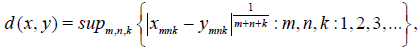(1)

Forall x = { xmnk } and y = { ymnk } in Γ3. Let φ = {finite sequences.

Consider a triple sequence x = (xmnk). The (m,n,k)th section x[m,n,k] of the sequence is defined by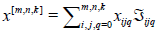for all m, n, k,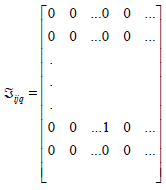with 1 in the (i, j, q)th position and zero otherwise.

A modulus function was introduced by Nakano . We recall that a modulus f is a function from [0,∞) →[0,∞), such that

(1) f (x) = 0 if and only if x = 0

(2) f (x+y) ≤ f (x)+ f (y,) for all x ≥ 0, y ≥ 0,

(3) f is increasing,

(4) f is continuous from the right at 0. Since | f (x) - f (y) |≤ f (x-y), it follows from here that f is continuous on [0,∞).

Let M and ? are mutually complementary Orlicz functions. Then, we have:

(i) For all u,y0,

uyM(u) + ?(y), (Young’s inequality)     (2)

(ii) For all u0, and 0 < λ < 1,

M (λu)λM (u)   (3)

Lindenstrauss and Tzafriri used the idea of Orlicz function to construct Orlicz sequence space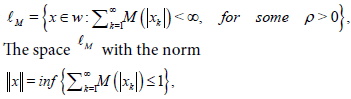becomes a Banach space which is called an Orlicz sequence space. For M(t)=tp (1≤ p < ∞),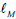coincide with the classical sequence space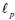.

A sequence f = (fmnk) of Orlicz function is called a Musielak -Orlicz function . A sequence g = (gmnk) defined by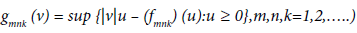is called the complementary function of a Musielak-Orlicz function f. For a given Musielak Orlicz function f, the Musielak-Orlicz sequence space tf.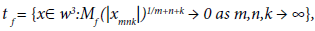where Mf is a convex modular defined by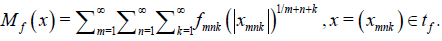We consider tf equipped with the Luxemburg metric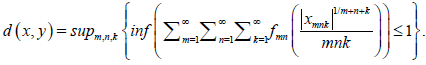#### Definition and Preliminaries

A sequence x = (xmnk) is said to be triple analytic if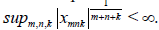The vector space of all triple analytic sequences is usually denoted by Λ3. A sequence x = (xmnk) is called triple entire sequence if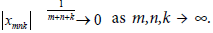The vector space of triple entire sequences is usually denoted by Γ3.

Let w3 denote the set of all complex double sequences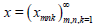and M :[0,∞) →[0,∞), be an Orlicz function. Given a triple sequence, xw3. Define the sets: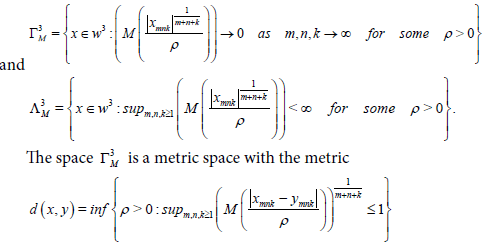Let n∈and X be a real vector space of dimension w, where nm. A real valued function

dp (x1,…,xn) =|| (d1(x1,0),…,dn(xn,0)) ||p on X satisfying the following four conditions:

(i) || (d1(x1,0),…,dn(xn,0)) ||p = 0 if and and only if d1(x1,0),…,dn(xn,0) are linearly dependent,

(ii) || (d1(x1,0),…,dn(xn,0)) ||p is invariant under permutation,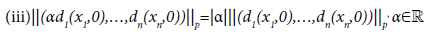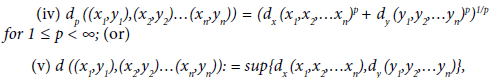for x1,x2,…xnX, y1,y2,…ynY is called the p product metric of the Cartesian product of n metric spaces is the p norm of the n - vector of the norms of the n subspaces.

A trivial example of p product metric of n metric space is the p norm space is X =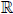equipped with the following Euclidean metric in the product space is the p norm: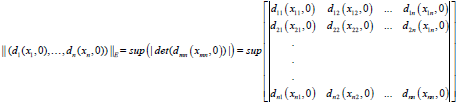Where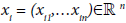for each i = 1,2,…n i =1, 2,…n.

If every Cauchy sequence in X converges to some LX, then X is said to be complete with respect to the p- metric. Any complete p- metric space is said to be p- Banach metric space.

Definition

A) Let X be a linear metric space. A function ρ: Xis called paranorm, if

(1) ρ (x)0, for all xX;

(2) ρ (-x) = ρ (x), for all xX;

(3) ρ (x+y)ρ (x) + ρ (y), for all x,yX;

(4) If (σmn) is a sequence of scalars with σmnσ as m,n → ∞ and (xmn) be a sequence of vectors with ρ (xmnx) → 0 as m,n → ∞, then ρ (σmnxmnσ x)→ 0 as m,n → ∞ [18,19].

A paranorm w for which ρ (x) = 0 implies x = 0 is called total paranorm and the pair (X,w) is called a total paranormed space. It is well known that the metric of any linear metric space is given by some total paranorm.

B) A family I⊂ 2Y×YY of subsets of a non empty set Y is said to be an ideal in Y if;

(1) φI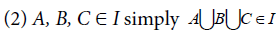(3) A, BI, CA imply CI.

while an admissible ideal I of Y further satisfies {x}∈I for each xY. Given I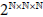be a non trivial ideal in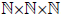A sequence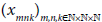in X is said to be I- convergent to 0 ∈ X, if for each ε > 0 the set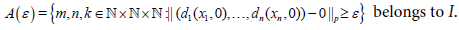C) A non-empty family of sets F2X×XX is a filter on X if and only if

(1) φF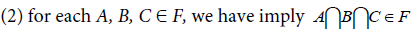(3) each A, BF and each BC, we have CF

D) An ideal I is called non-trivial ideal if Iφ and XI. Clearly I2X×XX is a non-trivial ideal if and only if F = F (1) = {X − A:A ∈ I} is a filter on X.

E) A non-trivial ideal I2X×XX is called (i) admissible if and only if {{x}:x ∈ X} ⊂ I. (ii) maximal if there cannot exists any non-trivial ideal J ≠ I containing I as a subset.

If we take I = If = {A ⊆: A is a finite subset}. Then If is a non-trivial admissible ideal ofand the corresponding convergence coincides with the usual convergence. If we take I = Iδ = { A ⊆: δ (A) = 0} where δ (A) denote the asymptotic density of the set A. Then Iδ is a non-trivial admissible ideal ofand the corresponding convergence coincides with the statistical convergence.

F) A sequence space E is said to be monotone if E contains the canonical pre-images of all its step spaces.

Remark

Let μ = (λrsu) be a non-decreasing sequence of positive real numbers tending to infinity and λ111 = 1 and r+1,s+1,u+1 ≤ λrsu + 1, for all r,s,u ∈.

The generalized de la Vallee-Poussin means is defined by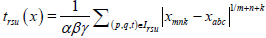Where Irsu = [r,s,u − λrsu + 1, rsu]. A sequence x = (xmnk) of complex numbers is said to be (V3)− summable to a number if trsu (x) → L as r,s,u → ∞.

#### Some New Integrated Statistical Convergence Sequence Spaces of pre-Cauchy

The main aim of this article to introduce the following sequence spaces and examine topological and algebraic properties of the resulting sequence spaces. Let p = (pmnk) be a sequence of positive real numbers for all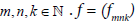be a sequence of Musielak-Orlicz function,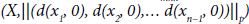be a p− metric space, and (αβγ) be a sequence of non-zero scalars and μmnk (X) = d(trsu,0) be a sequence of pre-Cauchy, we define the following sequence spaces as follows:

Definition

Let f is a Musielak Orlicz function and a triple sequence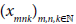is said to I− statistically convergent if, for any ε > 0 and δ > 0,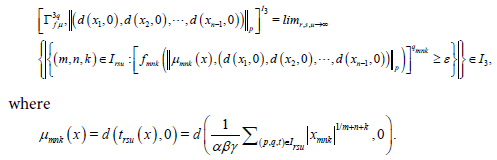#### Main Results

Theorem

Let f is a Musielak Orlicz function and if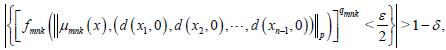for all (m,n,k) ∈ Ac, where c stands for the complement of the set A. Writing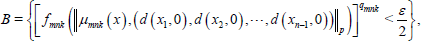we observe that m,n,k,a,b,cB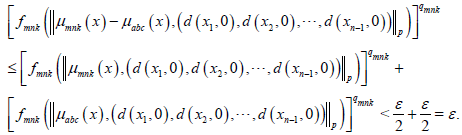Therefore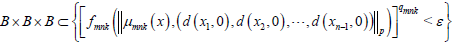which implies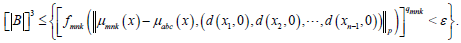Hence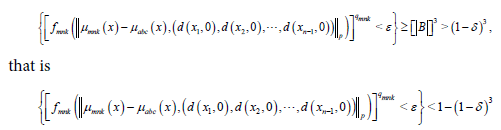for all (m,n,k)Ac. Let δ111 > 0 be given. Choosing δ > 0 so that 1−(1−δ)3 < δ111, we see that every (m,n,k)Ac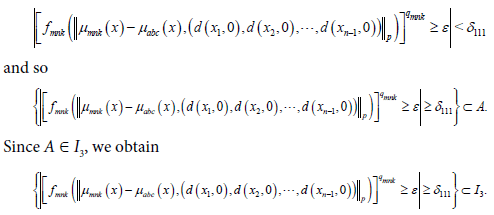Theorem

Let f is a Musielak Orlicz function and a triple sequence x = (xmnk) is I3 − statistically pre-cauchy if and only if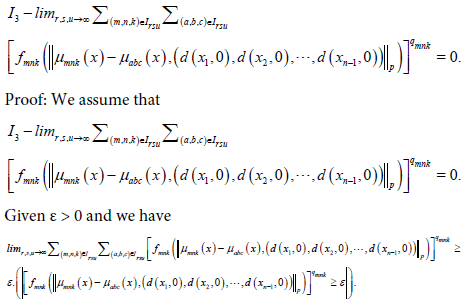Therefore for any δ > 0,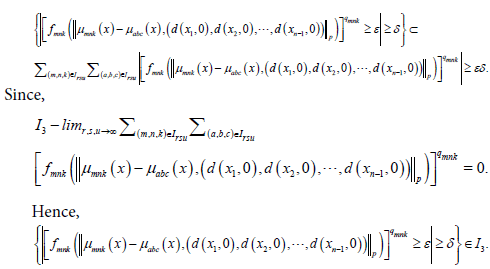Hence x is I3 statistically pre-Cauchy.

Conversely assume that x is I3, where I3 is triple sequence of ideal is statistically pre-cauchy and given ε > 0. Since x is analytic there exists an integer M such that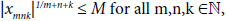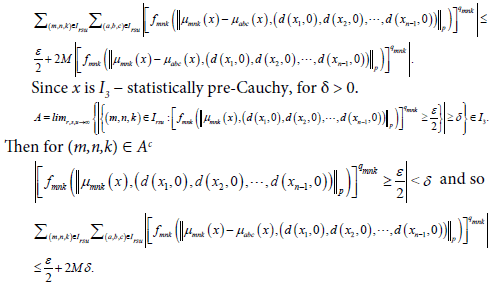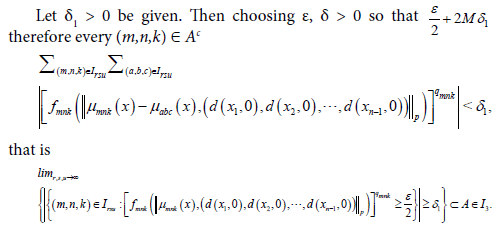This completes the proof.

#### Conclusion

We examine some properties of these concepts, if the triple entire sequence spaces is statistically convergent then statistically pre-Cauchy and also triple sequence of ideal is statistically pre-Cauchy. In future developed by rough statistical convergence on triple sequence and also rough sets in statistical convergence of fractional order of triple sequence of Γ.

#### Competing Interests

The authors declare that there is no conflict of interests regarding the publication of this research paper.

#### Acknowledgement

The authors are extremely grateful to the anonymous learned referee(s) for their keen reading, valuable suggestion and constructive comments for the improvement of the manuscript. The second author NS wish to thank the Department of Science and Technology, Government of India for the financial sanction towards this work under FIST program SR/FST/MSI-107/2015. The research of the first author Deepmala is supported by the Science and Engineering Research Board (SERB), Department of Science and Technology (DST), Government of India under SERB National Post-Doctoral fellowship scheme File Number: PDF/2015/000799. The authors are thankful to the editor(s) and reviewers of esteemed journal i.e., Advances in Robotics and Automation.

#### References

Select your language of interest to view the total content in your interested language

### Article Usage

• Total views: 1207
• [From(publication date):
December-2016 - Dec 09, 2019]
• Breakdown by view type
• HTML page views : 1115Can't read the image? click here to refresh